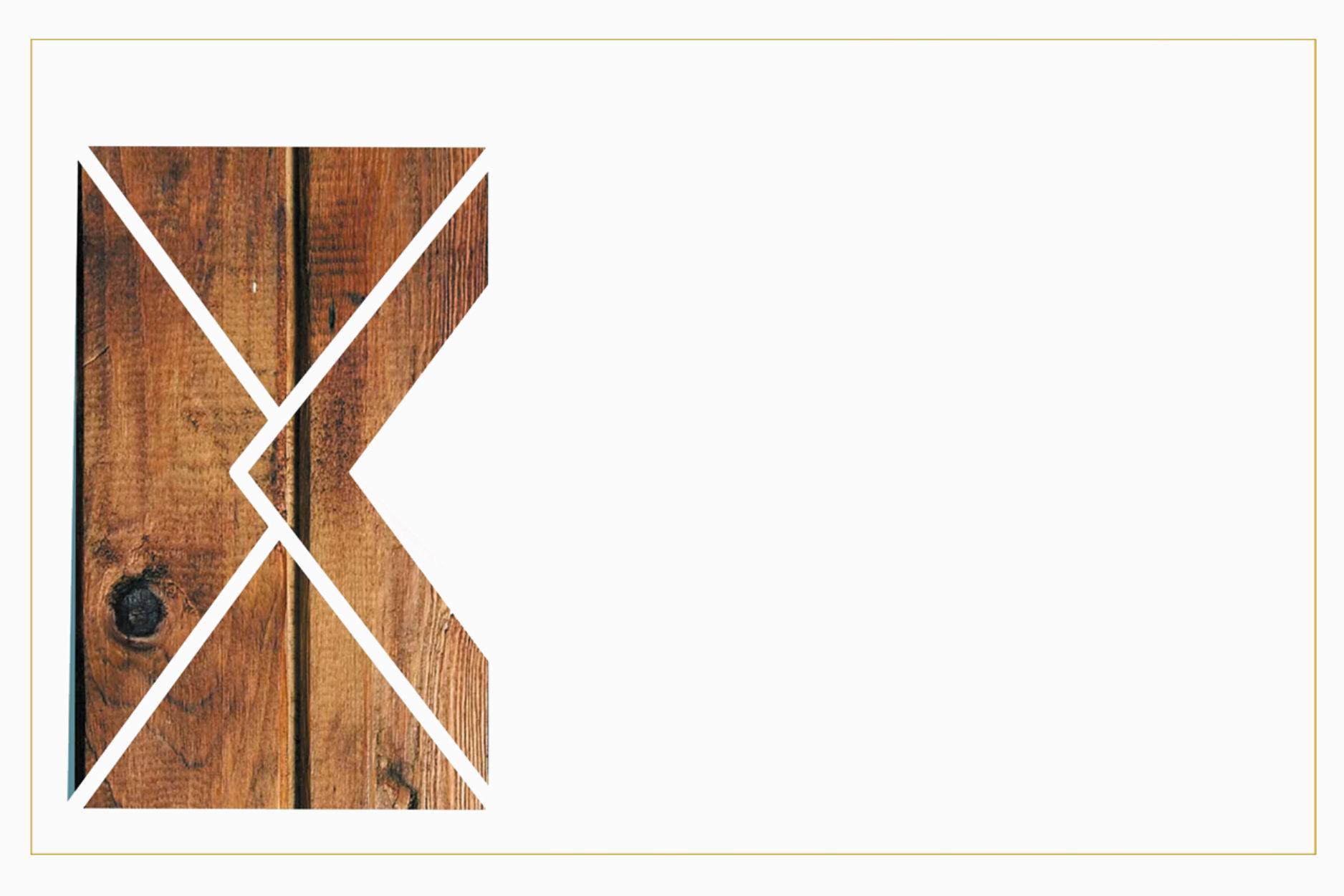### B A R  &  B R O T Z E I T

V O N   D O N N E R S T A G   B I S  S A M S T A G    G E Ö F F N E T !

Ö F F N U N G S Z E I T E N

D O   –   S A           A B        1 8   U H R

N E U R E U T H E R S T R.   21    |     8 0 7 9 9    M Ü N C H E N

N Ä H E   J O S E P H S P L A T Z     |     U 2

nachricht@derkleinekranich.deR E S E R V I E R E NS P E I S E N   &  G E T R Ä N K EM I E T E N+

I M P R E S S I O N E N